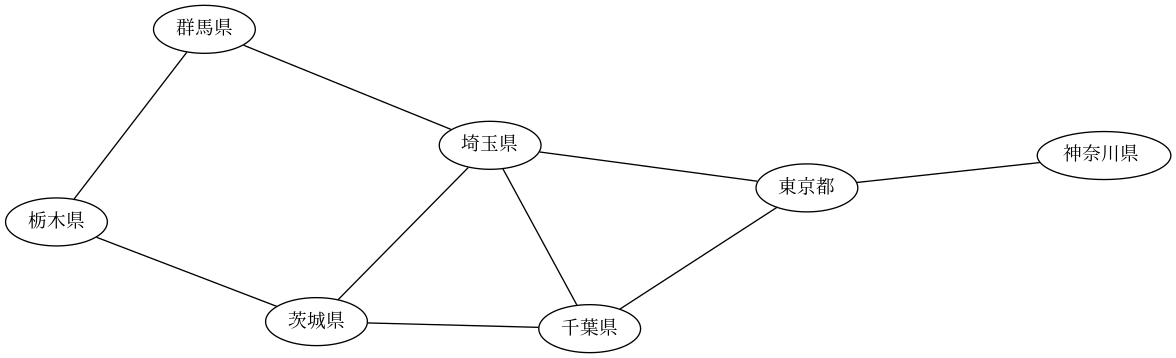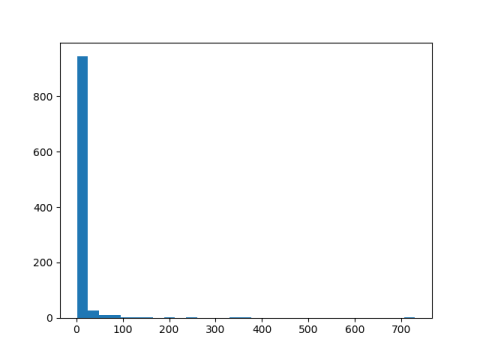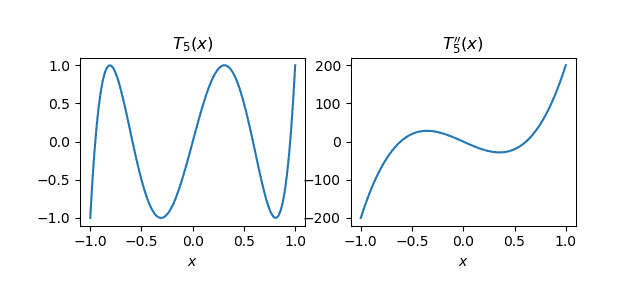# Expected value of X and 1/X

Yesterday I blogged about an exercise in the book The Cauchy-Schwarz Master Class. This post is about another exercise from that book, exercise 5.8, which is to prove Kantorovich’s inequality.

Assumeandfor non-negative numbers pi.

Thenwhereis the arithmetic mean of m and M andis the geometric mean of m and M.

In words, the weighted average of the x‘s times the weighted average of their reciprocals is bounded by the square of the ratio of the arithmetic and geometric means of the x‘s.

## Probability interpretation

I did a quick search on Kantorovich’s inequality, and apparently it first came up in linear programming, Kantorovich’s area of focus. But when I see it, I immediately think expectations of random variables. Maybe Kantorovich was also thinking about random variables, in the context of linear programming.

The left side of Kantorovich’s inequality is the expected value of a discrete random variable X and the expected value of 1/X.

To put it another way, it’s a relationship between E[1/X] and 1/E[X],which I imagine is how it is used in practice.

I don’t recall seeing this inequality used, but it could have gone by in a blur and I didn’t pay attention. But now that I’ve thought about it, I’m more likely to notice if I see it again.

## Python example

Here’s a little Python code to play with Kantorovich’s inequality, assuming the random values are uniformly distributed on [0, 1].

    from numpy import random

x = random.random(6)
m = min(x)
M = max(x)
am = 0.5*(m+M)
gm = (m*M)**0.5
prod = x.mean() * (1/x).mean()
bound = (am/gm)**2
print(prod, bound)


This returned 1.2021 for the product and 1.3717 for the bound.

If we put the code above inside a loop we can plot the product and its bound to get an idea how tight the bound is typically. (The bound is perfectly tight if all the x’s are equal.) Here’s what we get.All the dots are above the dotted line, so we haven’t found an exception to our inequality.

(I didn’t think that Kantorovich had made a mistake. If he had, someone would have noticed by now. But it’s worth testing a theorem you know to be true, in order to test that your understanding of the theorem is correct.)

# The baseball inequalityThere’s a theorem that’s often used and assumed to be true but rarely stated explicitly. I’m going to call it “the baseball inequality” for reasons I’ll get to shortly.

Suppose you have two lists of k positive numbers each:andThenThis says, for example, that the batting average of a baseball team is somewhere between the best individual batting average and the worst individual batting average.

The only place I can recall seeing this inequality stated is in The Cauchy-Schwarz Master Class by Michael Steele. He states the inequality in exercise 5.1 and gives it the batting average interpretation. (Update: This is known as the “mediant inequality.” Thanks to Tom in the comments for letting me know. So the thing in the middle is called the “mediant” of the fractions.)

Note that this is not the same as saying the average of a list of numbers is between the smallest and largest numbers in the list, though that’s true. The batting average of a team as a whole is not the same as the average of the individual batting averages on that team. It might happen to be, but in general it is not.

I’ll give a quick proof of the baseball inequality. I’ll only prove the first of the two inequalities. That is, I’ll prove that the minimum fraction is no greater than the ratio of the sums of numerators and denominators. Proving that the latter is no greater than the maximum fraction is completely analogous.

Also, I’ll only prove the theorem for two numerators and two denominators. Once you have proved the inequality for two numerators and denominators, you can bootstrap that to prove the inequality for three numerators and three denominators, and continue this process for any number of numbers on top and bottom.

So we start by assumingThen we have# Hadamard’s upper bound on determinant

For an n by n real matrix A, Hadamard’s upper bound on determinant iswhere aij is the element in row i and column j. See, for example, .

How tight is this upper bound? To find out, let’s write a little Python code to generate random matrices and compare their determinants to Hadamard’s bounds. We’ll take the square root of both sides of Hadamard’s inequality to get an upper bound on the absolute value of the determinant.

Hadamard’s inequality is homogeneous: multiplying the matrix A by λ multiplies both sides by λn. We’ll look at the ratio of Hadamard’s bound to the exact determinant. This has the same effect as generating matrices to have a fixed determinant value, such as 1.


from scipy.stats import norm
from scipy.linalg import det
import matplotlib.pyplot as plt
import numpy as np

# Hadamard's upper bound on determinant squared
return np.prod(np.sum(A**2, axis=1))

N = 1000
ratios = np.empty(N)

dim = 3
for i in range(N):
A = norm.rvs(size=(dim, dim))

plt.hist(ratios, bins=int(N**0.5))
plt.show()



In this simulation the ratio is very often around 25 or less, but occasionally much larger, 730 in this example.It makes sense that the ratio could be large; in theory the ratio could be infinite because the determinant could be zero. The error is frequently much smaller than the histogram might imply since a lot of small values are binned together.

I modified the code above to print quantiles and ran it again.

    print(min(ratios), max(ratios))
qs = [0.05, 0.25, 0.5, 0.75, 0.95]
print( [np.quantile(ratios, q) for q in qs] )


This printed

    1.0022 1624.9836
[1.1558, 1.6450, 2.6048, 5.7189, 32.49279]


So while the maximum ratio was 1624, the ratio was less than 2.6048 half the time, and less than 5.7189 three quarters of the time.

Hadamard’s upper bound can be very inaccurate; there’s no limit on the relative error, though you could bound the absolute error in terms of the norm of the matrix. However, very often the relative error is moderately small.

 Courant and Hilbert, Methods of Mathematical Physics, Volume 1.

# Convex function of diagonals and eigenvalues

Sam Walters posted an elegant theorem on his Twitter account this morning. The theorem follows the pattern of an equality for linear functions generalizing to an inequality for convex functions. We’ll give a little background, state the theorem, and show an example application.

Let A be a real symmetric n×n matrix, or more generally a complex n×n Hermitian matrix, with entries aij. Note that the diagonal elements aii are real numbers even if some of the other entries are complex. (A Hermitian matrix equals its conjugate transpose, which means the elements on the diagonal equal their own conjugate.)

A general theorem says that A has n eigenvalues. Denote these eigenvalues λ1, λ2, …, λn.

It is well known that the sum of the diagonal elements of A equals the sum of its eigenvalues.We could trivially generalize this to say that for any linear function φ: RR,because we could pull any shifting and scaling constants out of the sum.

The theorem Sam Walters posted says that the equality above extends to an inequality if φ is convex.Here’s an application of this theorem. Assume the eigenvalues of A are all positive and let φ(x) = – log(x). Then φ is convex, andand soi.e. the product of the diagonals of A is an upper bound on the determinant of A.

This post illustrates two general principles:

1. Linear equalities often generalize to convex inequalities.
2. When you hear a new theorem about convex functions, see what it says about exp or -log.

# Sum and mean inequalities move in opposite directions

It would seem that sums and means are trivially related; the mean is just the sum divided by the number of items. But when you generalize things a bit, means and sums act differently.

Let x be a list of n non-negative numbers,and let r > 0 [*]. Then the r-mean is defined to beand the r-sum is define to beThese definitions come from the classic book Inequalities by Hardy, Littlewood, and Pólya, except the authors use the Fraktur forms of M and S. If r = 1 we have the elementary mean and sum.

Here’s the theorem alluded to in the title of this post:

As r increases, Mr(x) increases and Sr(x) decreases.

If x has at least two non-zero components then Mr(x) is a strictly increasing function of r and Sr(x) is a strictly decreasing function of r. Otherwise Mr(x) and Sr(x) are constant.

The theorem holds under more general definitions of M and S, such letting the sums be infinite and inserting weights. And indeed much of Hardy, Littlewood, and Pólya is devoted to studying variations on M and S in fine detail.

Here are log-log plots of Mr(x) and Sr(x) for x = (1, 2).Note that both curves asymptotically approach max(x), M from below and S from above.

## Related posts

[*] Note that r is only required to be greater than 0; analysis books typically focus on r ≥ 1.

# The Brothers Markov

The Markov brother you’re more likely to have heard of was Andrey Markov. He was the Markov of Markov chains, the Gauss-Markov theorem, and Markov’s inequality.

Andrey had a lesser known younger brother Vladimir who was also a mathematician. Together the two of them proved what is known as the Markov Brothers’ inequality to distinguish it from (Andrey) Markov’s inequality.

For any polynomial p(x) of degree n, and for any non-negative integer k, the maximum of the kth derivative of p over the interval [-1, 1] is bounded by a constant times the maximum of p itself. The constant is a function of k and n but is otherwise independent of the particular polynomial.

In detail, the Markov Brothers’ inequality saysAndrey proved the theorem for k = 1 and his brother Vladimir generalized it for all positive k.

The constant in the Markov Brothers’ inequality is the smallest possible because the bound is exact for Chebyshev polynomials .

Let’s look at an example. We’ll take the second derivative of the fifth Chebyshev polynomial.

T5(x) = 16x5 – 20x3 + 5x.

The second derivative is

T5”(x) = 320x3 – 120x.

Here are their plots:The maximum of T5(x) is 1 and the maximum of its second derivative is 200.

The product in the Markov Brothers’ inequality with n = 5 and k = 2 works out to

(25/1)(24/3) = 200

and so the bound is exact for p(x) = T5(x).

***

It took a while for westerners to standardize how to transliterate Russian names, so you might see Andrey written as Andrei or Markov written as Markoff.

There were even more ways to transliterate Chebyshev, including Tchebycheff, Tchebyshev, and Tschebyschow. These versions are the reason Chebyshev polynomials  are denoted with a capital T.

 There are two families of Chebyshev polynomials. When used without qualification, as in this post, “Chebyshev polynomial” typically means Chebyshev polynomial of the first kind. These are denoted Tn. Chebyshev polynomials of the second kind are denoted Un.

# Bounding the 3rd moment by the 4th moment

For a random variable X, the kth moment of X is the expected value of Xk.

For any random variable X with 0 mean, or negative mean, there’s an inequality that bounds the 3rd moment, m3 in terms of the 4th moment, m4:The following example shows that this bound is the best possible.

Define

u = (1 – √ 3)/√ 2
v = (1 + √ 3)/√ 2
p = (3 + √ 3) / 6

and suppose Xu with probability p and Xv with probability q = 1 – p. Then X has mean 0, and you can show that exact equality holds in the inequality above.

Here’s some Mathematica code to verify this claim.

    u = (1 - Sqrt)/Sqrt
v = (1 + Sqrt)/Sqrt
p = (Sqrt + 1)/(2 Sqrt)
q = 1 - p
m3 = p u^3 + q v^3
m4 = p u^4 + q v^4
Simplify[ (4/27)^(1/4) m4^(3/4) / m3 ]


As hoped, the code returns 1.

Reference: Iosif Pinelis, Relations Between the First Four Moments, American Mathematical Monthly, Vol 122, No 5, pp 479-481.

# Gaussian correlation inequality

The Gaussian correlation inequality was proven in 2014, but the proof only became widely known this year. You can find Thomas Royan’s remarkably short proof here.

Let X be a multivariate Gaussian random variable with mean zero and let E and F be two symmetric convex sets, both centered at the origin. The Gaussian correlation inequality says that

Prob(in E and F) ≥ Prob(X in E) Prob(X in F).

Here’s a bit of Python code for illustrating the inequality. For symmetric convex sets we take balls of p-norm r where p ≥ 1 and r > 0. We could, for example, set one of the values of p to 1 to get a cube and set the other p to 2 to get a Euclidean ball.

from scipy.stats import norm as gaussian

def pnorm(v, p):
return sum( abs(x)**p for x in v )**(1./p)

def simulate(dim, r1, p1, r2, p2, numReps):
count_1, count_2, count_both = (0, 0, 0)
for _ in range(numReps):
x = gaussian.rvs(0, 1, dim)
in_1 = (pnorm(x, p1) < r1)
in_2 = (pnorm(x, p2) < r2)
if in_1:
count_1 += 1
if in_2:
count_2 += 1
if in_1 and in_2:
count_both += 1
print("Prob in both:", count_both / numReps)
print("Lower bound: ", count_1*count_2 * numReps**-2)

simulate(3, 1, 2, 1, 1, 1000)


When numReps is large, we expect the simulated probability of the intersection to be greater than the simulated lower bound. In the example above, the former was 0.075 and the latter 0.015075, ordered as we’d expect.

If we didn’t know that the theorem has been proven, we could use code like this to try to find counterexamples. Of course a simulation cannot prove or disprove a theorem, but if we found what appeared to be a counterexample, we could see whether it persists with different random number generation seeds and with a large value of  numReps. If so, then we could try to establish the inequality analytically. Now that the theorem has been proven we know that we’re not going to find real counterexamples, but the code is only useful as an illustration.

# Functional means

Functional means are a way of inducing a mean Mf from a differentiable function f.If f(x) = x2, Mf(x, y) = (x + y)/2 is the arithmetic mean.

If f(x) = 1/x, Mf(x, y) = √(xy) is the geometric mean.

Seems like this should be useful for something.

Source

Related post: Means and inequalities

# Three books on inequalities

The classic book on inequalities is Inequalities by Hardy, Littlewood, and Pólya, first published in 1934 (ISBN 0521358809). I’ve mentioned it a few times before. The book is quite thorough. I imagine more people use it as a reference than read it cover to cover.The Cauchy-Schwarz Master Class by Michael Steele (ISBN 052154677X) is a more recent book on inequalities, published in 2004. The preface explains what the title means by a “master class.”

In the fine arts, a master class is a small class where students and coaches work together to support a high level of technical and creative excellence. This book tries to capture the spirit of a master class while providing coaching for readers who want to refine their skills as solvers of problems, especially those problems dealing with mathematical inequalities.

The Cauchy-Schwarz Master Class book lives up to its stated aim. Reading the book and working through the exercises reminds me of taking music lessons. The emphasis is on learning techniques rather than cataloging specific results.The final book I’ll mention is When Less is More: Visualizing Basic Inequalities by Claudi Alsina and Roger B. Nelsen (ISBN 0883853426). Like The Cauchy-Schwarz Master Class, this book emphasizes problem solving technique. More specifically, it emphasizes geometric techniques for understanding and proving inequalities. (I’ve written a review of When Less is More, but unfortunately you have to log in as an MAA member to read it.) When Less is More is as concerned with beauty as with truth.You can tell from the cover art and the architectural allusion in the title that this book cares about aesthetics. Each proof is a polished gem.